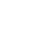Published on

# JavaScript for vs forEach vs map | JS array methodsAuthors
•Name
Gyanendra Kumar Knojiya
@gyanknojiya

The distinction between a forEach and a for loop is explained in-depth in this article. The key distinctions between the two are listed below.

## 1. for loop

The JavaScript for loop is used to iterate over an array of items for a set number of times. It should be utilized if a particular number of iteration is known.

for (initial_value; condition; increment) {
// code to be perform
}


#### Example

// table of 5
for (let i = 1; i <= 10; i++{
console.log(5 * i);
}
// output
5
10
15
20
25
30
35
40
45
50


## 2. forEach loop

The forEach() method loops across arrays as well, but it does it in a different way than the standard “for loop.” It passes the following arguments along with a callback function for each entry of an array:

• Current Value (required): The current array element’s value
• index (optional): The current element’s index number
• array (Optional): This is the array object to which the current element belongs.

Using the forEach method, we require a callback function to loop through an array.

#### Example

// Array of all days
const days = [
“Sunday”,
“Monday”,
“Tuesday”,
“Wednesday”,
“Thursday”,
“Friday”,
“Saturday”,
];

days.forEach(function (day) {
console.log(day);
});


The function will be run for each and every element in the array. At least one parameter in the callback should reflect the items of an array.

#### Output

Sunday
Monday
Tuesday
Wednesday
Thursday
Friday
Saturday


#### Syntax

forEach((element) => { ... } )
forEach((element, index) => { ... } )
forEach((element, index, array) => { ... } )


## 3. map loop

The map() method populates a new array with the results of performing a function on each member of the calling array.

#### Example

const numbers = [1, 3, 4, 5, 7, 6, 2, 4]
const twoTimes = numbers.map((num) => num * 2)


It will loop over all of the elements in the array, multiply them by two, and return an array.

#### Output

;[2, 6, 8, 10, 14, 12, 4, 8]


Syntax

map((element) => { ... })
map((element, index) => { ... })
map((element, index, array) => { ... })